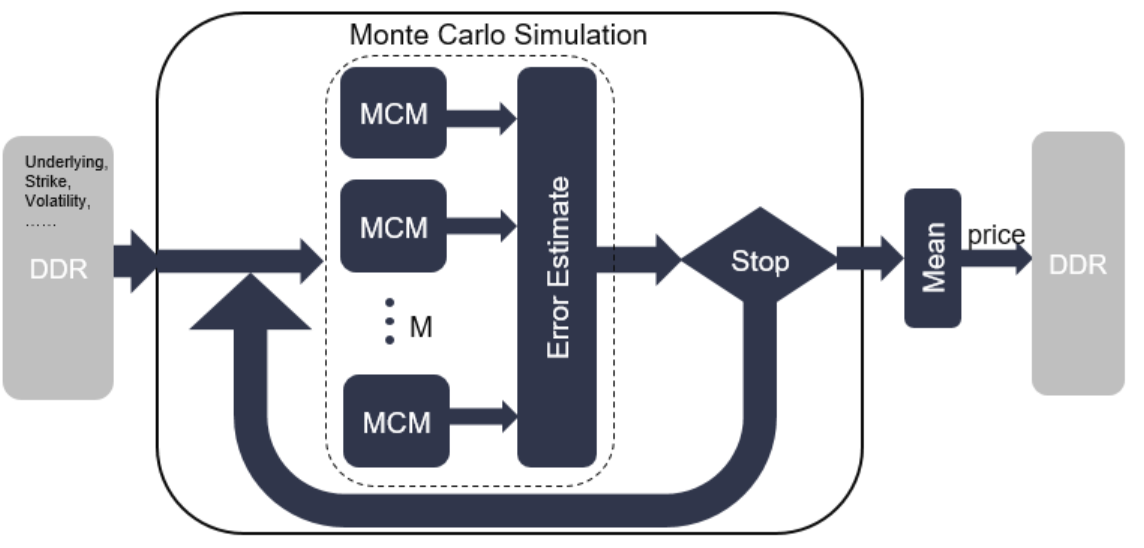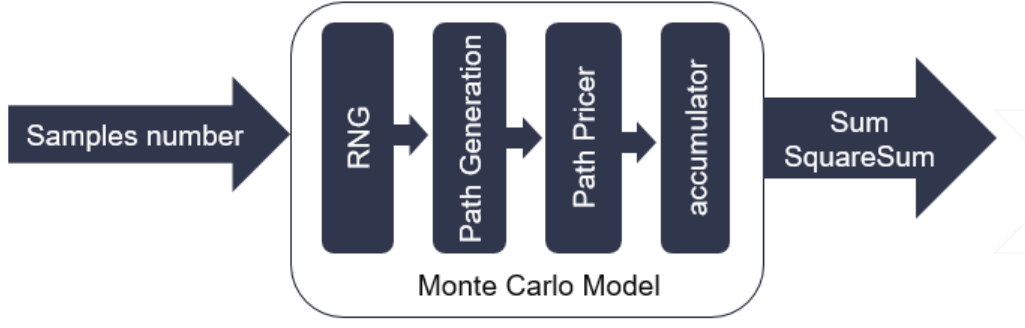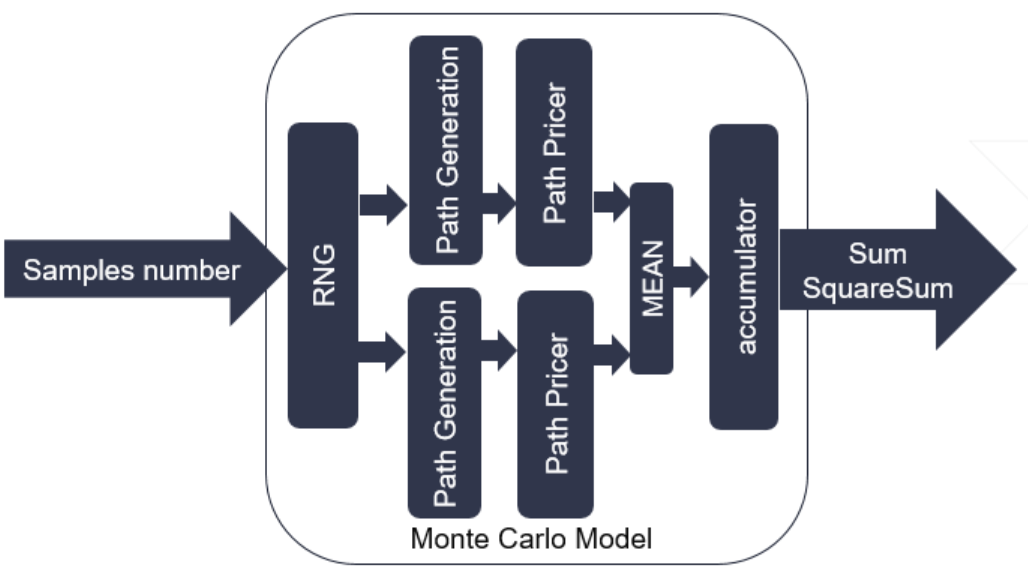# Monte Carlo Simulation¶

## Overview¶

The essence of Monte Carlo Method is the law of large numbers. It uses statistics sampling to approximate the expectation.

It simulates the stochastic process of value of underlying asset. When using Monte Carlo to price the option, the simulation generates a large amount of price paths for underlying asset and then calculates the payoff based on the associated exercise style. These payoff values are averaged and discounted to today. The result is the value to the option today.

Most of the time, the value of underlying asset are affected by multiple factors, and it don’t have a theoretical analytic solution. For this scenario, Monte Carlo Methods are very suitable.

## Framework¶

The framework of Monte Carlo Simulations is as follows. The top module Monte Carlo Simulation will call the Monte Carlo Module (MCM) multiple times until it reaches the required samples number or required tolerance.Every MCM generates a batch of paths. The number of MCM (M) is a template parameter, the maximum of which is related to the FPGA resource. Each MCM includes an RNG module, a path generator module, a path pricer module, and an accumulator. All of these modules are in dataflow region and connected with hls::stream.RNG module generates the normal random numbers. Currently, only generating pseudo-random number is supported. The detailed implementation of RNG inside may refer to the RNG section.

Path Generator uses the random number to calculate the price paths of underlying asset. Currently, Black-Scholes and Heston valuation model are supported.

Path pricer will exercise the option based on the price paths of underlying asset and calculate the payoff, discount the payoff to time zero for option value. Different option has associated implementation for path pricer.

Accumulator sums together the option value and square of option value on all the paths. These sums are prepared for calculation of average and variance. Because the accumulation of floating point data type cannot achieve II = 1, the input is dispatched to 16 sub-accumulator and sum the result of 16 sub-accumulator at last.## Antithetic paths¶

Antithetic paths is a kind of variance reduction techniques.

The precision of Monte Carlo Simulation is related with the simulations times. The error of results is an order of O($$\frac{1}{\sqrt{N}}$$).

If $$X$$ applies to $$\phi(0,1)$$, then the antithetic variable of is $$-X$$. We can call $$X$$ and $$-X$$ as an antithetic pair. In our implementation, when the antithetic template parameter is set to true. The RNG module will generate two random number at one clock cycles. Then, two path generators are followed to make sure it can consume two random number at on clock cycles. At the same time, the two price paths are averaged at path pricer. The structure with antithetic is as follows.

The advantage of antithetic paths is not only reducing the number of generated random number from 2N to N, but also reduces the variance of samples paths and improves the accuracy if the correlation of two antithetic variables is negative.